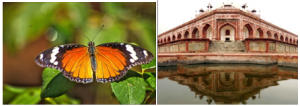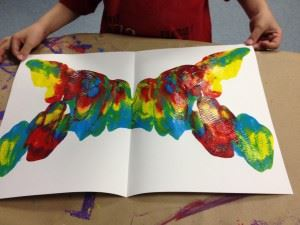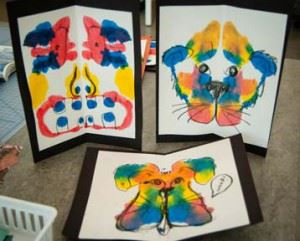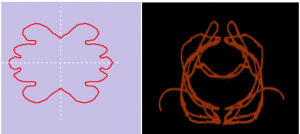# Figures with Symmetry

We see symmetry all around us, structure of monuments, bodies of insects, human skeletal system, man made objects and patterns etc. Ever wondered how the intricate symmetrical patterns were formed by architectures and engineers in the magnificent masterpieces we see every day. In this section, we will learn about the simple techniques to make interesting symmetric figures.## Making Symmetric Figures

Ink Blot Figures: Symmetric figures can be made very easily. Following are the steps we follow to make an inkblot symmetric figure.

• We fold a piece of paper into two halves along the centre crease.
• We spill drops of ink or watercolour in our desired pattern on one half of the sheet
• We gently press both the halves together and rub all around the folded paper.

This method results in a symmetric pattern where the fold of the paper is the line of symmetry, an example of which is shown below.In the above figure, we folded the paper along the vertical line, parallel to the width, we can also fold the paper horizontally along the length or diagonally, the line of symmetry will vary accordingly.### Symmetry of Inked String Figures

An inked string can also be used to make symmetric figures. Following steps are followed to make an inked string figure.

• We fold a piece of paper into two halves along the centre crease.
• We arrange strings dipped in variety of colors in desired pattern on one half of the paper.
• We gently press both the halves together and rub all around the folded paper.In the above figure, we folded the paper along the vertical line, parallel to the width. We can also fold the paper horizontally along the length or diagonally, the line of symmetry will vary accordingly.